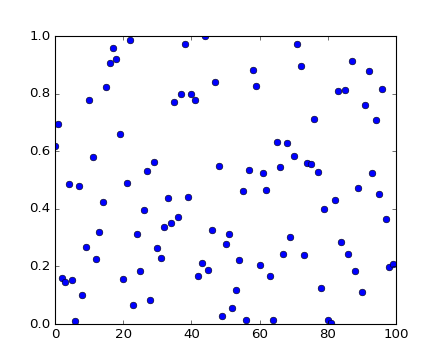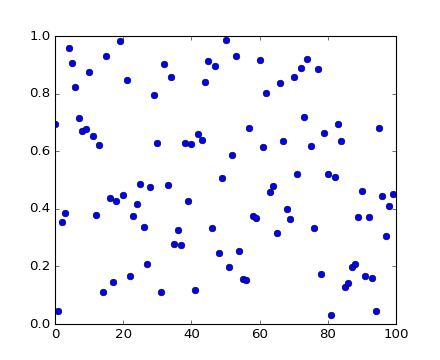## Matplotlib 2.0.0rc2 is available

Install the release candidate now!Travis-CI:# pylab_examples example code: shared_axis_across_figures.py¶(png, hires.png, pdf)(png, hires.png, pdf)

```"""
connect the data limits on the axes in one figure with the axes in
another.  This is not the right way to do this for two axes in the
same figure -- use the sharex and sharey property in that case
"""
import numpy as np
import matplotlib.pyplot as plt

fig1 = plt.figure()
fig2 = plt.figure()

ax1.plot(np.random.rand(100), 'o')
ax2.plot(np.random.rand(100), 'o')

# In the latest release, it is no longer necessary to do anything
# special to share axes across figures:

# ax1.sharex_foreign(ax2)
# ax2.sharex_foreign(ax1)

# ax1.sharey_foreign(ax2)
# ax2.sharey_foreign(ax1)

plt.show()
```

Keywords: python, matplotlib, pylab, example, codex (see Search examples)